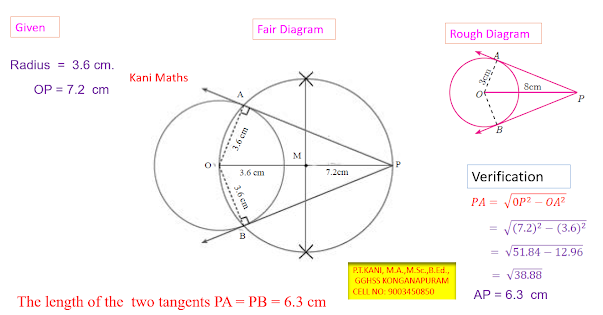# Tamil Nadu Board Class 10 Solutions for Mathematics –  Practical Geometry (English Medium)

STD - 10 : CHAPTER - 4  PRACTICAL GEOMETRY English Medium Published By : P.Thirukumaresakani M.A.,M.Sc.,B.Ed., GGHSS, KONGANAPURAM, SALEM DT.

The study of Geometry is concerned with knowing properties of various shapes and

structures. Arithmetic and Geometry were considered to be the two oldest branches of

mathematics. Greeks held Geometry in high esteem and used its properties to discuss

various scientific principles which otherwise would have been impossible. Eratosthenes

used the similarity of circle to determine the circumference of the Earth, distances

of the moon and the sun from the Earth, to a remarkable accuracy. Apart from these

achievements, similarity is used to find width of rivers, height of trees and much more.

Geometry plays vital role in the field of Science, Engineering and Architecture. We

see many Geometrical patterns in nature. We are familiar with triangles and many of their

properties from earlier classes.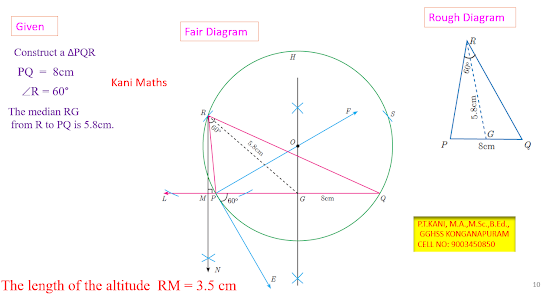Ex 4.10 Construct a triangle similar to a given triangle PQR with its sides equal to of the corresponding sides of the triangle PQR (scale factor < 1).

Ex 4.11 Construct a triangle similar to a given triangle PQR with its sides equal to of the corresponding sides of the triangle PQR (scale factor > 1).

EXERCISE - 4.1

10. Construct a triangle similar to a given triangle PQR with its sides equal to  of the corresponding sides of the triangle PQR. (Scale factor <1).

11. Construct a triangle similar to a given triangle LMN with its sides equal to  of the corresponding sides of the triangle LMN. (Scale factor  <1).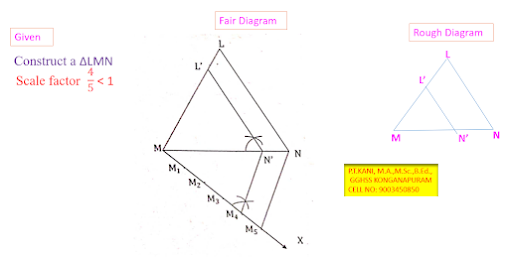12. Construct a triangle similar to a given triangle ABC with its sides equal to  of the corresponding sides of the triangle ABC. (Scale factor   >1).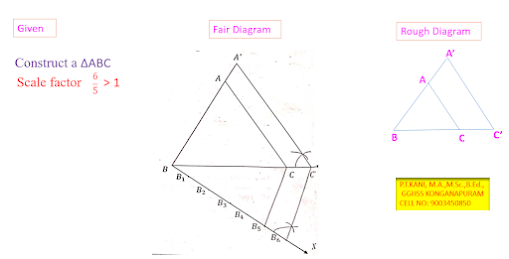13. Construct a triangle similar to a given triangle PQR with its sides equal  to of the corresponding sides of the triangle PQR. (Scale factor   >1).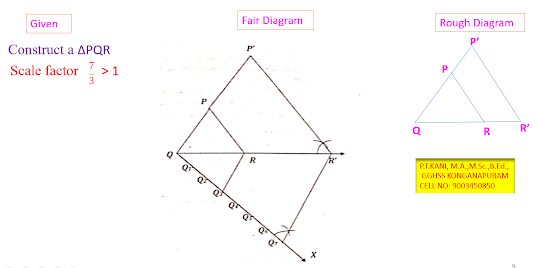Example 4.17  : Construct a ΔPQR in which PQ=8cm, ÐR=60°and the median RG from R to PQ is 5.8cm.Find the length of the altitude from R to PQ.

Example 4.18 :  Construct a triangle ΔPQR such that QR=5cm, ÐP=30° and the altitude from P to QR is oflength 4.2 cm.

Ex 4.19 Draw a triangle ABC of base BC=8cm, ÐA=60° and the bisector of ÐA meets BC at D suchthat BD=6cm.

EXERCISE - 4.2

11. Construct a ΔPQR which the base PQ=4.5cm, ÐR=35° and the median from R to RG is 6cm.

12. Construct a ΔPQR in which QR=5cm, ÐP=40° and the median PG from P to QR is 4.4cm. Find the length of the altitude from P to QR.

13. Construct a ΔPQR such that QR=6.5cm, ÐP=60° and the altitude from P to QR is of length 4.5cm.

14. Construct a ΔABC such that AB=5.5cm, ÐC=25° and the altitude from C to AB is 4cm.

15. Draw a triangle ABC of base BC=5.6cm, ÐA=40° and the bisector of A meets BC at D such that CD=4cm.

16. Draw ΔPQR such that PQ=6.8cm, vertical angle is 50° and the bisector of the vertical angle meets the base at D where PD=5.2cm.

EXERCISE - 4.3

14. Draw the two tangents form a point which is 10cm away from the centre of a a circle of radius 5cm.Also, measure the lengths of the tangents.

15. Take a point which is 11cm away from the centre of a circle of radius 4cm and draw the two tangents to the circle from that point.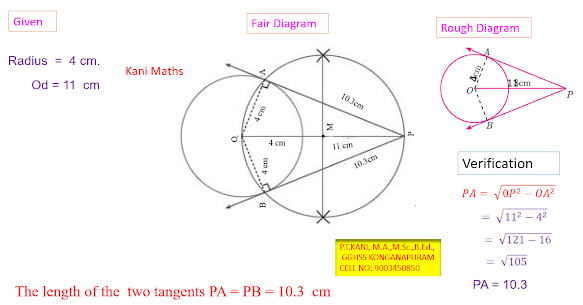16. Draw the two tangents from a point which is 5cm away from the centre of a circle of diameter 6cm. Also, measure the length of the tangents.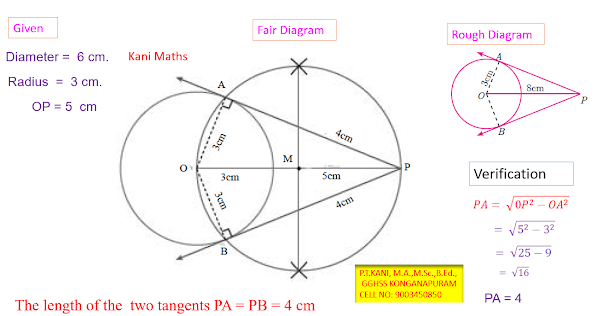17. Draw the tangents to the circle from the point p having radius 3.6cm , and centre at 0. Point P is at a distance 7.2cm from the centre.